# 6. A sphere of negligible size has a charge of +190 nC and is placed at the origin of a coordinate system.a. Sketch the sphere with electric field lines and equipotential lines.b. What is the electric field (magnitude and direction) and the electric potential at the point (2 cm, 0)and at the point (0,1 cm) due to the charge?c. If a proton is placed at the points in part a. what is the force (magnitude and direction) on and thepotential energy of the proton?d. Repeat part c with an electron instead of a proton.

Question
114 views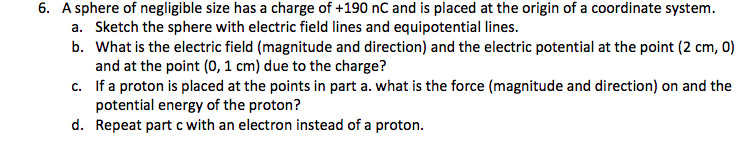help_outlineImage Transcriptionclose6. A sphere of negligible size has a charge of +190 nC and is placed at the origin of a coordinate system. a. Sketch the sphere with electric field lines and equipotential lines. b. What is the electric field (magnitude and direction) and the electric potential at the point (2 cm, 0) and at the point (0,1 cm) due to the charge? c. If a proton is placed at the points in part a. what is the force (magnitude and direction) on and the potential energy of the proton? d. Repeat part c with an electron instead of a proton. fullscreen
check_circle

Step 1

(a)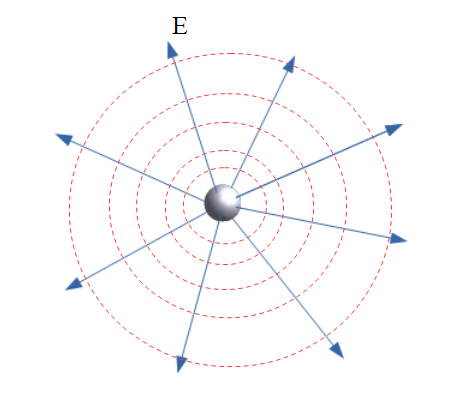E is the direction of the electric field, since the charge on the particle is positive so, the direction of the electric field is away from the charge as shown in the figure.

Red dashed lines are equipotential surfaces. The charge is point-like, so, distribution of the electric field in the space would be symmetrical. So, the shape of the equipotential surface must be symmetrical (spherical) as shown in the figure.

Step 2

(b)

Given quantities,

Charge on the particle,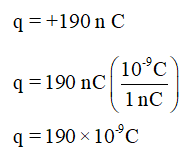The distance of the point where the electric field has to find,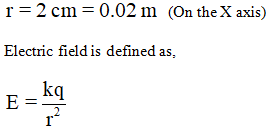Let’s plug all values, we get,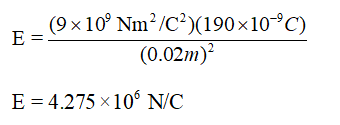The charge is positive, so, the direction of the electric field would be away from the charged particle. According to the question, the charge is on the x-axis, so, the direction of the electric field would be along the positive direction of x.

Electric potential is defined as,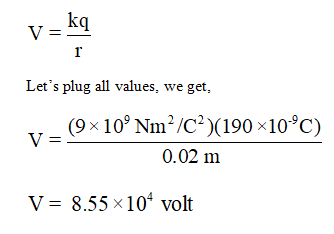Another location of the point where electric field and potential have to find,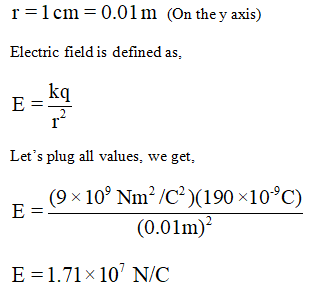The direction of the electric field would be along the positive direction of y.

Now, the electric potential is defined as,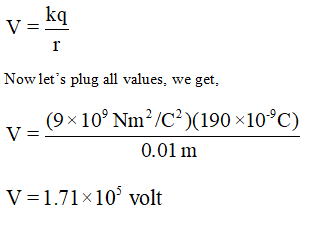Step 3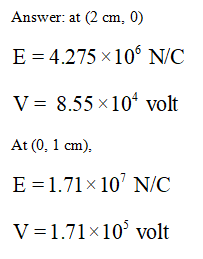...

### Want to see the full answer?

See Solution

#### Want to see this answer and more?

Solutions are written by subject experts who are available 24/7. Questions are typically answered within 1 hour.*

See Solution
*Response times may vary by subject and question.
Tagged in